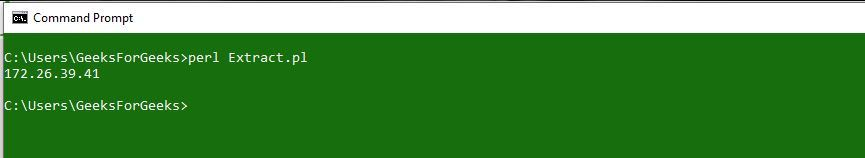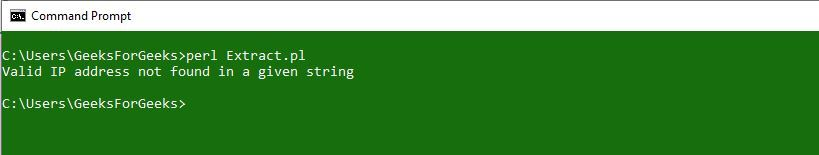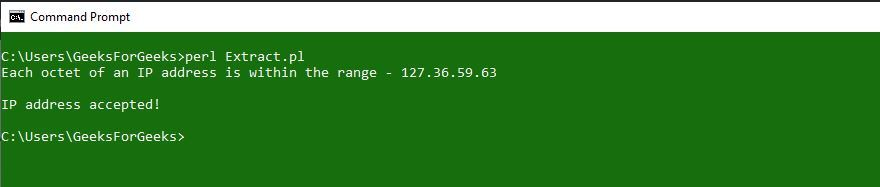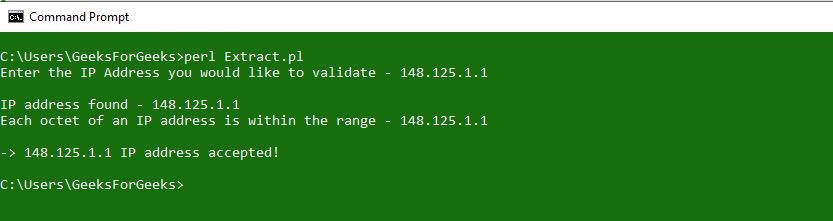# Perl | Extract IP Address from a String using Regex

Perl stands for Practical Extraction and Reporting Language and this not authorized acronym. One of the most powerful features of the Perl programming language is Regular Expression and in this article, you will learn how to extract an IP address from a string. A regular expression can be either simple or complex, depending on the pattern you want to match like our title – Extracting IP Address from a String using Regex. Extracting IP address from a string can be a simple or challenging task. Hence, people love and hate regular expressions. They are a great way to express a simple pattern and a horrific way to express complicated ones. Some of the examples of Quantifiers as well as Characters and their meaning is given below:

Quantifier Meaning
a* zero or more a’s
a+ one or more a’s
a? zero or one a’s
a{m} exactly m a’s
a{m,} at least m a’s
a{m,n} at least m but at most n a’s
Character Meaning
^ beginning of string
\$ end of string
. any character except newline
* match 0 or more times
+ match 1 or more times
? match 0 or 1 times
| alternative
( ) grouping
[ ] set of characters
{ } repetition modifier
\ quote or special

### Extracting an IP Address from a String

The easiest way is just to take any string of four decimal numbers separated by periods is

```\d{1,3}\.\d{1,3}\.\d{1,3}\.\d{1,3})/ OR /^\d+\.\d+\.\d+\.\d+\$/
```

In the following example we simply extracting an IP address from a given string.

 `#!/usr/bin/perl ` ` `  `my` `\$ip` `= ``"MY IP ADDRESS IS172.26.39.41THIS IS A VALID IP ADDRESS"``; ` ` `  `if``(``\$ip` `=~ /(\d{1,3}\.\d{1,3}\.\d{1,3}\.\d{1,3})/) ` `{ ` `    ``\$ip` `= \$1; ` `    ``print` `"\$ip\n"``; ` `} `

Output:But, the above example also accepts the wrong IP address like 596.368.258.269. We know, a proper decimal dotted IP address has no value larger than 255 and writing a regex that matches the integers 0 to 255 is hard work as the regular expressions don’t understand arithmetic; they operate purely textually. Therefore, you have to describe the integers 0 through 255 in purely textual means.

Now, we will see an implementation of extracting an IP address which will check octet range also.

 `#!/usr/bin/perl ` ` `  `my` `\$ip` `= ``"MY IP ADDRESS IS 36.59.63 THIS IS A VALID IP ADDRESS"``; ` ` `  `if``(``\$ip` `=~ /(\d{1,3}\.\d{1,3}\.\d{1,3}\.\d{1,3})/) ` `{ ` `    ``if``(\$1 <= 255 && \$2 <= 255 && \$3 <= 255 && \$4 <= 255) ` `    ``{ ` `        ``print``(``"Each octet of an IP address "``, ` `              ``"is within the range - \$1\n"``); ` `        ``print``(``"\nIP address accepted!\n"``); ` `    ``} ` `    ``else` `    ``{ ` `        ``print``(``"Octet not in range - \$1\n"``, ` `              ``"IP address not accepted\n"``); ` `    ``} ` `} ` `else` `{ ` `    ``print``(``"Valid IP address not found in a given string\n"``); ` `} `

Output:If you change the string to

`[my \$ip = "MY IP ADDRESS IS 127.36.59.63 THIS IS A VALID IP ADDRESS";] `

then the output isIn the following example, we are accepting a string from the user which contains an IP address and then we are extracting the IP address from it. We used the chomp() function to remove any newline character from the end of a string.

 `#!/usr/bin/perl ` ` `  `print``(``"Enter the IP Address you would like to validate - "``); ` `my` `\$ip` `= ; ` ` `  `if``(``\$ip` `=~ /(\d{1,3}\.\d{1,3}\.\d{1,3}\.\d{1,3})/) ` `{ ` `    ``\$ip` `= \$1; ` `} ` ` `  `chomp``(``\$ip``); ` ` `  `if``(``\$ip` `=~ m/^(\d\d?\d?)\.(\d\d?\d?)\.(\d\d?\d?)\.(\d\d?\d?)\$/) ` `{ ` `    ``print``(``"\nIP address found - \$ip\n"``); ` `    ``if``(\$1 <= 255 && \$2 <= 255 && \$3 <= 255 && \$4 <= 255) ` `    ``{ ` `        ``print``(``"Each octet of an IP address is "``, ` `              ``"within the range - \$1.\$2.\$3.\$4\n"``); ` `        ``print``(``"\n-> \$ip IP address accepted!\n"``); ` `    ``} ` `    ``else` `    ``{ ` `        ``print``(``"Octet(s) out of range. "``, ` `              ``"Valid number range between 0-255\n"``); ` `    ``} ` `} ` `else` `{ ` `    ``print``(``"IP Address \$ip is not in a valid format\n"``); ` `} `

Output:My Personal Notes arrow_drop_upIf you like GeeksforGeeks and would like to contribute, you can also write an article using contribute.geeksforgeeks.org or mail your article to contribute@geeksforgeeks.org. See your article appearing on the GeeksforGeeks main page and help other Geeks.

Please Improve this article if you find anything incorrect by clicking on the "Improve Article" button below.

Article Tags :
Practice Tags :

Be the First to upvote.

Please write to us at contribute@geeksforgeeks.org to report any issue with the above content.